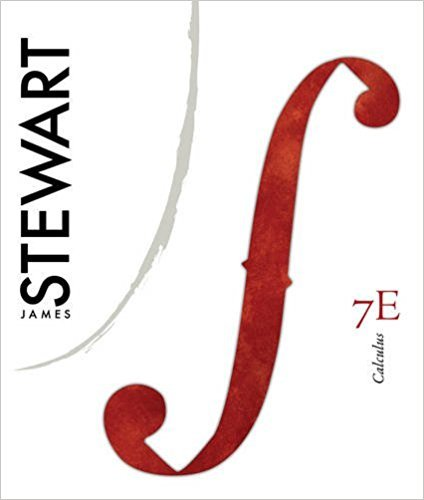×
Get Full Access to Calculus, - 7 Edition - Chapter 2 - Problem 59
Get Full Access to Calculus, - 7 Edition - Chapter 2 - Problem 59

×

# Suppose that and , where , , , , and . Find (a) and (b) .ISBN: 9780538497817 125

## Solution for problem 59 Chapter 2

Calculus, | 7th Edition

• Textbook Solutions
• 2901 Step-by-step solutions solved by professors and subject experts
• Get 24/7 help from StudySoup virtual teaching assistantsCalculus, | 7th Edition

4 5 1 402 Reviews
19
3
Problem 59

Suppose that and , where , , , , and . Find (a) and (b) .

Step-by-Step Solution:
Step 1 of 3

Step 2 of 3

Step 3 of 3

##### ISBN: 9780538497817

Calculus, was written by and is associated to the ISBN: 9780538497817. The answer to “Suppose that and , where , , , , and . Find (a) and (b) .” is broken down into a number of easy to follow steps, and 16 words. The full step-by-step solution to problem: 59 from chapter: 2 was answered by , our top Calculus solution expert on 11/10/17, 05:23PM. Since the solution to 59 from 2 chapter was answered, more than 278 students have viewed the full step-by-step answer. This full solution covers the following key subjects: Find, suppose, Where. This expansive textbook survival guide covers 17 chapters, and 887 solutions. This textbook survival guide was created for the textbook: Calculus,, edition: 7.

Unlock Textbook Solution